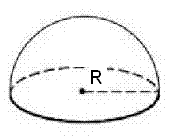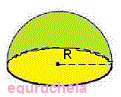# Hemisphere Calculator

Calculate the volume, curved surface area(CSA) and total surface area(TSA) of hemisphere.
Volume of Hemisphere: [ (2/3)πr³ ]
 Enter the Radius : Volume of Hemisphere =

Curved Surface Area(CSA) of Hemisphere: [ 2πr² ]
 Enter the Radius : Curved Surface Area(CSA) of Hemisphere =

Total Surface Area(TSA) of Hemisphere: [ 3πr² ]
 Enter the Radius : Total Surface Area(TSA) of Hemisphere =## Formula

TSA of Hemisphere: [ 3πr² ]

CSA of Hemisphere: [ 2πr² ]

Volume of Hemisphere: [ (2/3)πr³ ]

### Hemispherical cylinder volume

The Surface Area of Hemisphere is the area occupied by the surface of the solid object and it is classified into two types as follows:

1. Curved Surface Area (CSA)

2. Total Surface Area (TSA)Curved surface area (CSA)

The Curved Surface Area of Hemisphere is the half part of a sphere, therefore the curved surface area is also half of that sphere.

CSA = 1/2 ( 4 π r2) = 2 π r2

Area of base

The Base Area is the base of the hemisphere in circular shape. As we know by the formula of area of circle as follows:

Area of base = π r2

Total Surface Area (TSA)

To calculate the Total Surface Area of the hemisphere, we need to consider the base of the hemisphere which is circular. Therefore, it is equal to:

TSA = Curved Surface Area + Area of the Base Circle

= 2 π r2 + π r2

= 3 π r2